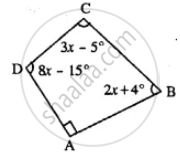# Use the Information Given in the Following Figure to Find : (I) X (Ii) ∠B and ∠C - Mathematics

Sum

Use the information given in the following figure to find :

(i) x
(ii) ∠B and ∠C#### Solution

∵ ∠A = 90°    (Given)

∠B = (2x + 4°)

∠C = (3x - 5°)

∠D = (8x - 15°)

∠A + ∠B + ∠C + ∠D = 360°

90° + (2x + 4°) + (3x - 5°) + (8x - 15°) = 360°

90° + 2x + 4° + 3x - 5° + 8x - 15° = 360°

⇒ 74° + 13x = 360°

⇒ 13x = 360° - 74°

⇒ 13x = 286°

⇒ x = 22°

∵ ∠B = 2x  4 = 2 × 22° + 4 = 48°

∠C = 3x - 5 = 3 × 22° - 5 = 61°

Hence (i) 22° (ii) ∠B = 48°, ∠C = 61°

Is there an error in this question or solution?

#### APPEARS IN

Selina Concise Mathematics Class 8 ICSE
Chapter 16 Understanding Shapes
Exercise 16 (C) | Q 4 | Page 187
Selina Class 6 Mathematics